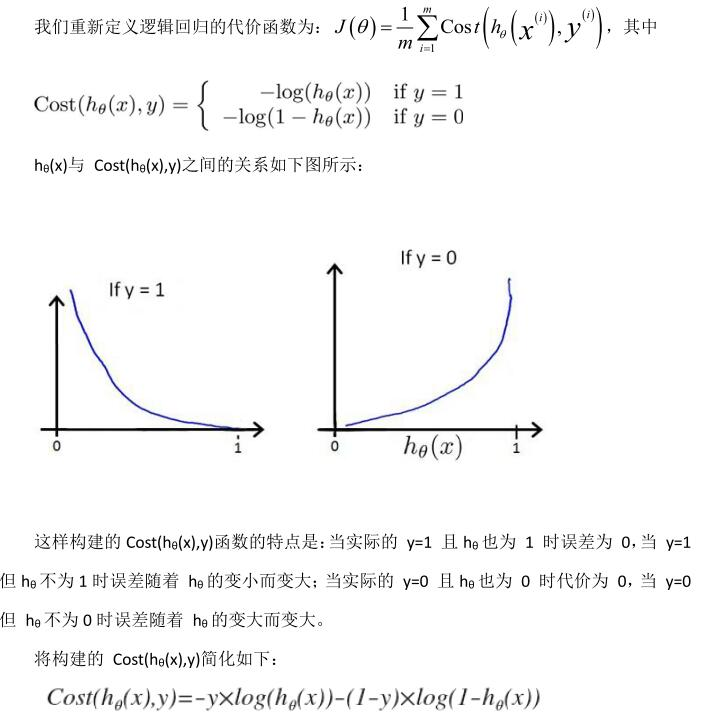yiˆ=θxi

C=i=1n(yiyiˆ)2

# 二、逻辑回归的损失函数

P(X=n)={p1p,n=1,n=0

## 1.对数损失函数的形式

L(Y,P(Y|X))=logP(Y|X)$L(Y, P(Y|X)) = -\log P(Y|X)$这个对数损失函数的意思是指分类为Y的情况下，使P(Y|X)达到最大。若模型是用最大概率的分类来做预测的，而Y是代表分类为正确的分类，而P(Y|X)则是代表正确分类的概率，那对数取反就是P(Y|X)$P(Y|X)$越大，损失函数就越小。P(Y|X)=1$P(Y|X)=1$时，损失就降为0，不可能再低了。

L(θ)=i=1mP(y=1|xi)yiP(y=0|xi)1yi

logL(θ)=i=1m[yilogP(y=1|xi)+(1yi)log(1P(y=1|xi))]

L(Y,P(Y|X))=logP(Y|X)=yilogP(Y|X)+(1yi)log(1P(Y|X))## 2.直接根据对数损失函数的形式得到目标函数

P(X=y|x)=hθ(x)=g(f(x))=11+exp(f(x))1hθ(x)=1g(f(x))=exp(f(x))1+exp(f(x)),y=1,y=0

L(Y,P(Y|X))=log(Y|X)

L(y,P(Y=y|X))={log(hθ(x))log(1hθ(x)),y=1,y=0

J(θ)=1mi=1m[yilog(hθ(xi))+(1yi)log(1hθ(xi))]